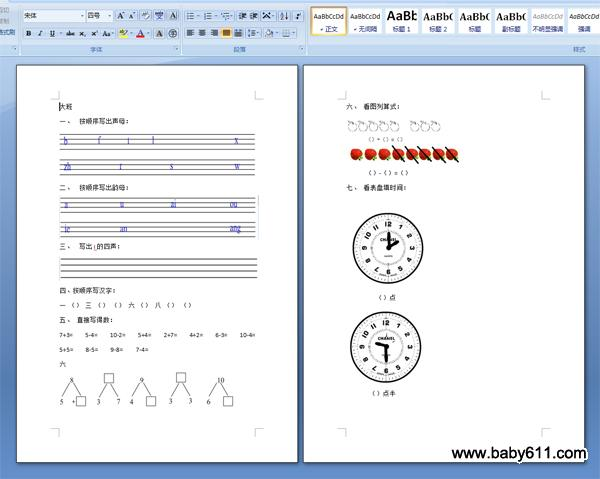幼儿园大班平时测试题大班

一、按顺序写出声母：

二、按顺序写出韵母：

三、写出i的四声：

四、按顺序写汉字：

一 （） 三 （） （） 六 （） 八 （） （）

五、直接写得数：

7+3=   5-4=   10-2=   5+4=   2+7=   4+2=   6-3=   10-4=  5+5=    8-5=    9-8=    7-4=

六

六、看图列算式：

（）+（）=（）

（）-（）=（）

七、看表盘填时间：

（）点

（）点半

• 幼儿园试卷分类
• 将此试卷分享到：
• 大班试卷最新更新